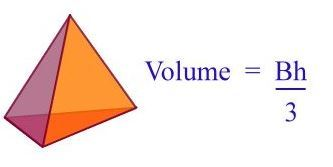Volume of Pyramids
4 years ago
murphyll
Save
Edit
Host a game
Live GameLive
Homework
Solo Practice
Practice15 QuestionsShow answers
• Question 1
180 secondsQ. Find the volume of the figure.
1,800 cm³
900 cm³
600 cm³
750 cm³
• Question 2
180 secondsQ. Find the volume of the figure.
126 cm3
907.5 cm3
605 cm3
55 cm3
• Question 3
30 seconds
Q. What is the formula for the VOLUME of a PYRAMID?
1/2pl
4/3(pi)r3
1/3Bh
1/3(pi)r2h
• Question 4
180 secondsQ. Find the volume of the figure. Round to the nearest whole number.
144 cm³
288 cm³
48 cm³
24 cm³
• Question 5
30 seconds
Q. Find the volume of a square pyramid with the side length 3cm  and height of 5cm.
60cm3
75cm3
45cm3
15cm3
• Question 6
60 secondsQ. On a trip to Oregon, Sabrina bought a small stone in the shape of a square pyramid as a souvenir. Determine the volume of the stone. Round to the nearest tenth.
4.5 in ³
6 in ³
6.75 in ³
1.5 in ³
• Question 7
30 seconds
Q. To find the volume of a triangular prism, you must first find the area of the triangular base then multiply by the height of the prism finally, dividing by 3.
True
False
• Question 8
180 secondsQ. Lucia has a triangular prism that has a length of 6cm and the width of 4cm and the height is 8cm. What is the volume
96cm³
54cm³
80cm³
24cm³
• Question 9
30 secondsQ. The Toblerone candy come in a package like the triangular prism. The bases of the triangles are 2 inches with a height of 2.5 inches. The faces are 10 inches in length.
What is the volume of the package?
25 cubic in.
8 1/3 cubic in.
50 cubic in.
12.5 cubic in.
• Question 10
30 secondsQ. The shape of the base in a rectangular prism is a rectangle.
True
False
• Question 11
30 seconds
Q. What is the equation you use to find the volume of a rectangular pyramid?
V= 1/2 (bh)
V= 1/3 (bh)
V= 1/3(base)(length)(height)
V= 1/2(base)(length)(height)
• Question 12
30 seconds
Q. To find the volume of a rectangular pyramid, you must first find the area of the rectangular base then multiply by the height of the pyramid finally, dividing by 3.
True
False
• Question 13
30 secondsQ. The area of the base of this rectangular prism can be found by using which equation?
B = 8x9
B = 8 x 8
B = 1/3 (8 x 8)
B = 1/3 (8 x 9)
• Question 14
30 secondsQ. Which equation can be used to find the volume of this figure?
Vol = (8 x 8 x 9) (1/3)
Vol = (8 x 9) ( 8)
Vol = (1/3 x 8 x 9) (9)
Vol = (1/2 x 8 x 9) (8)
• Question 15
180 secondsQ. Find the volume of this figure?